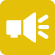## 1-Minute Survey

What Type of Investor Are You? – Take This 1-Min Survey to Find Out

Financial Literacy

# What is Straight-Line Depreciation?

When companies invest in assets, they expect those assets to last a certain number of years. Over time, they’re depreciated based on their remaining serviceable life and any potential saleable value left in them. Done consistently over a period of years, it creates a linear expense model, known as straight-line depreciation.

Companies calculate straight-line depreciation by subtracting the salvage value from the asset’s cost and dividing it by the number of years estimated for its useful life. It’s one of four depreciation calculations and the simplest for many companies to use, since the depreciable amount remains the same. Graphed as asset value over years, this depreciation occurs as a straight line—hence its name.

Here’s a look at how companies use straight-line depreciation and how it’s factored into enterprise asset management.## A Look at Tangible Assets

Depreciation in any form only applies to tangible capital assets. These are assets a company can see and touch, which serve to facilitate vital business operations. They can include machinery, equipment, buildings, vehicles and much, much more—anything carried on the balance sheet for more than one year (fixed).

The type of asset is important for predicting its depreciation, since original acquisition cost matters. Some assets hold their value better than others over time, which can affect the useful life expectancy of the asset, as well as its salvage value.

## Calculating Straight-Line Depreciation

The formula for straight line depreciation includes three important variables: asset price, useful life and salvage value

• Asset price is the total amount paid for the asset.
• Useful life is the number of years the asset will continue to generate income.
• Salvage value is the amount the asset will sell for after its useable life.

In the case of straight-line depreciation, the amount depreciated is the same year over year. This simplifies the equation. The formula for calculating straight-line depreciation is:

Annual Depreciation Amount = (Asset Price – Salvage Value) / Useful Life

For example, ABC Company might buy a service vehicle for \$50,000. The company expects the vehicle to offer five years of useful life and a salvage value of \$5,000 after it’s retired. The annual depreciation amount for the vehicle would be \$9,900 per year.

## Straight-Line vs. Other Types of Depreciation

Straight-line depreciation is one of four modes of asset depreciation businesses can use. The others include:

• Declining balance
• Sum-of-years digits
• Units of production

What’s the difference between these methods and straight-line? Straight-line allows companies to expense the same amount annually. This simplifies accounting and asset life cycle management. The other methods are “accelerated modes” of depreciation, which allow companies to depreciate assets much quicker, to minimize taxable income. Each is a viable option for depreciating assets; the best strategy depends on the objectives of the company.

## How Does Straight-Line Depreciation Affect Accounting?

Depreciation is a tricky subject when it comes to accounting. The way companies choose to depreciate assets can impact the balance sheet and change the way certain accounts look, for better or for worse. Thankfully, straight-line depreciation is easy to contextualize, since it features a static number that’s easily lifted out of calculations.

For example, a company might make a \$1 million investment in equipment with a useful life of 10 years. Assuming no salvage cost, it would depreciate \$100,000 each year. This would appear on the company’s financial statements as a credit (to offset the asset), thus, raising the company’s bottom line. This can reflect positively on the company’s performance and cause its share price to rise, even if there’s no change in operational figures.

Companies carrying a balance sheet heavy in assets tend to have significant depreciation figures, often staggered over different periods. Even straight-line depreciation is difficult to keep track of. That’s why Net Asset Value (NAV) is a favorite valuation metric of investors seeking to understand a company’s value, sans depreciation.

## Be Mindful of Assumptions

Like any forward-looking estimation, depreciation involves assumptions. To calculate the annual depreciation amount, companies make two distinct assumptions: useful life and salvage value. However, there’s no guarantee either figure is accurate at the time of estimation. Companies may need to adjust depreciation based on changing variables in an asset’s life or changes in salvage value. For this reason, many will often assume a zero-dollar salvage value to simplify the calculation.

Another important consideration regarding assumptions involves posturing. Sometimes, companies will inflate useful life or salvage value in an attempt to create a more favorable depreciation schedule. This can help bolster investor support in the near-term. For example, a company investing in a \$600,000 IT infrastructure may estimate a useful life at 12 years and a salvage value of \$80,000. Meanwhile, it’s like that the tech will become antiquated in eight years and sell for \$25,000.

## Linear Depreciation is Predictable

Many companies choose a straight-line model for depreciation because it’s predictable. In fact, expending the same amount for an asset each year makes it easier to predict how that depreciation will show up in accounting—and what to expect from an asset over the next X number of years. Similarly, it’s easy for investors to see and understand how asset depreciation affects the company’s financials.

Therefore, to continue expanding your investment and financial knowledge, sign up for the Investment U e-letter below. You will discover valuable stock insights and financial literacy education from some of the nation’s top analysts and Wall Street experts!

Linear depreciation—like any method of expending an asset—is subject to assumptions. However, calculated responsibly, these assumptions can be accurate and reliable. As part of a straight-line depreciation model, they’re useful figures in understanding exactly how a company sees its assets and the role those assets play in generating income.